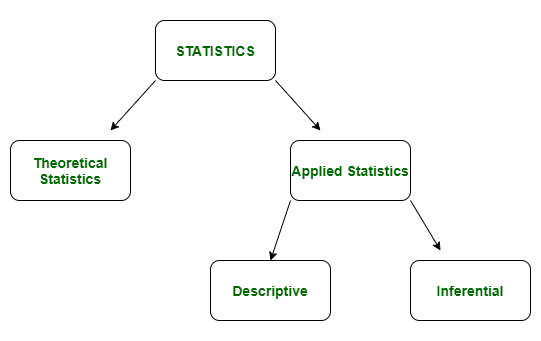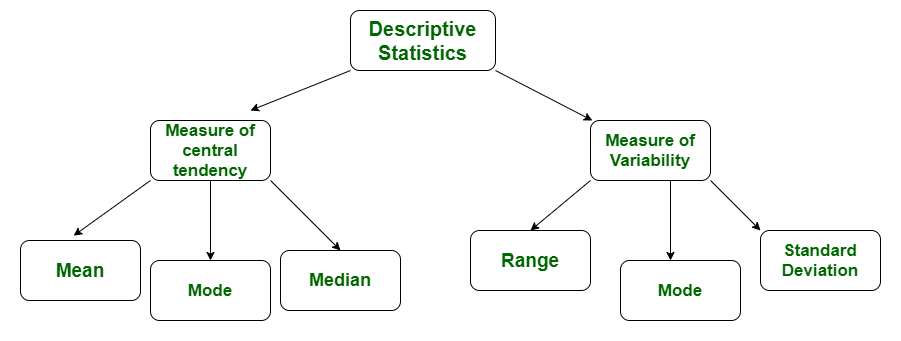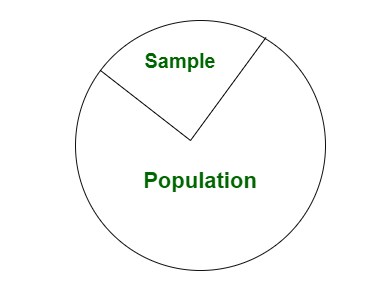# Difference between Descriptive and Inferential statistics

• Difficulty Level : Medium
• Last Updated : 21 Aug, 2020

Statistics is a branch of mathematics dealing with the collection, analysis, interpretation, and presentation of masses of numerical data. It is basically a collection of quantitative data.

Types of Statistics –

• Theoretical Statistics
• Applied Statistics1. Descriptive Statistics :
Descriptive statistics is a term given to the analysis of data that helps to describe, show and summarize data in a meaningful way. It is a simple way to describe our data. Descriptive statistics is very important to present our raw data ineffective/meaningful way using numerical calculations or graphs or tables. This type of statistics is applied on already known data.

Types of Descriptive Statistics –

• Measure of Central Tendency
• Measure of Variability2. Inferential Statistics :
In inferential statistics predictions are made by taking any group of data in which you are interested. It can be defined as a random sample of data taken from a population to describe and make inference about the population. Any group of data which includes all the data you are interested is known as population. It basically allows you to make predictions by taking a small sample instead of working on whole population.Difference between Descriptive and Inferential statistics :

S.No. Descriptive Statistics Inferential Statistics
1. It gives information about raw data which describes the data in some manner. It makes inference about population using data drawn from the population.
2. It helps in organizing, analyzing and to present data in a meaningful manner. It allows us to compare data, make hypothesis and predictions.
3. It is used to describe a situation. It is used to explain the chance of occurrence of an event.
4. It explain already known data and limited to a sample or population having small size. It attempts to reach the conclusion about the population.
5. It can be achieved with the help of charts, graphs, tables etc. It can be achieved by probability.

My Personal Notes arrow_drop_up
Recommended Articles
Page :